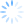Would you like to use CDXZipStream across a variety of applications, such as Microsoft Access, Word, Powerpoint, or Sharepoint?  With a little programming knowledge it’s actually quite easy to use Visual Basic to make a “data call” to CDXZipStream, get the data you need and put it in the application of choice.  This allows you to grab CDXZipstream data without entering a custom function formula into a worksheet cell. There are also other advantages in that this approach can be faster for very large sets of data, and since the values are entered directly into the cells, there are no delays associated with Excel or any other application recalculating a lot of formulas.  (That’s one of the reasons we use this approach in our series of one-click templates.)

Let’s see how this works with a very simple example using the CDXZipStream function CDXZipCode.  This right-click function in Excel takes a zip code and returns a host of associated data for the zip, such as city, population, elevation. latitude, longitude, etc.  (The data fields vary depending upon the CDXZipStream version.) The worksheet custom function formula to get the city for the zip code 07869 would be:

= CDXZipCode ("07869", "City")

But we can also do the same thing using just a few lines of Visual Basic code.  First, in a Visual Basic module we use the createobject statement to connect to CDXZipStream:

Then simply ask for the data:

The code can be generalized to obtain data for a long list of zip codes.  In Microsoft Excel, for example, just loop through the worksheet range containing the list of zips:

For N = 1 to 100

Zip_code = Sheets(1).Range(“A” & N & “”)   ‘Get each zip code from the worksheet list

Sheets(1).Range(“B” & N & “”) = City           ‘Place the returned data in the worksheet next to its corresponding zip code

Next N

Let’s take a look at another programming example that can do something a little more complex: performing a radius analysis for addresses based on their geocoded (latitude and longitude) locations.

(Note:  If you'd like to do this analysis without using code, see our blog article How to Very Accurately Filter Addresses Based on Distance.)

To get latitude and longitude for an address, you can use the worksheet function CDXLocateMP with the following custom function formulas:

latitude = CDXLocateMP(1,"123 Main Street","Springfield","OH","45501")

longitude = CDXLocateMP(2,"123 Main Street","Springfield","OH","45501")

To do this in Visual Basic, again use the createobject statement to connect to CDXZipStream:

and then get the data:

You can design the code to loop through all the addresses, assigning new values for the variables Address, City, State, and PostalCode for each data set.  Then use the returned latitude and longitude data to calculate the distance to a target address that has also been geocoded, using the function CDXDistance2WP:

After looping through all the address pairs, just sort or filter the distance data to find the ones that are closest to the target location. This is a very fast calculation, and is much more accurate than typical zip code radius calculations that use the centroid of the zip code area.

In our last example, we will perform route optimization for a set of driving stops, which will  illustrate how to deal with data arrays for both the input and output of a CDXZipStream function.   Using the normal custom function formula in Excel, the worksheet formula would be something like this, using the CDXRouteMP:

=CDXRouteMP(0,8,A1:A10)

In this case we are requesting the quickest route (the first parameter, "0'), and the list of optimized stops or waypoints on the route (the second parameter, "8") as the output. We also list the worksheet range of input destinations that are located in cells A1 through A10.

In Visual Basic, start with the createobject statement to connect to CDXZipStream:

Instead of using a worksheet range, we need to define a VB array that contains all of the destinations on the route.  We can just loop through the worksheet, like this:

For N = 1 to 10

Input_array(N) = Sheets(1).Range("A" & N & "")

Next N

Use the newly defined Input_array to get the optimized list of stops: